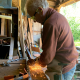# Scaling Up the Size of the Yurt

I want to talk about scaling up the size of the yurt. We had considered adding this in the book but decided against it. So I will take you through the process now. The size or diameter of the yurt in the book was 16 feet. Considering the next reasonable size up one might think of a 20-foot diameter. Let’s follow the steps to creating it. The parts that get affected are the foundation, floor panels, wall panels (though not height), beam length, roof panels, and possibly the skylight (size). If you follow this process you can create a yurt of any size (within reason, as loads on the beams become a factor). We begin with the floor plan, which in turn informs us of what the foundation will be.

With a diameter of 20 feet we are looking for the circumference. That is found with the formula C = d X pi (circumference = diameter X 3.1416). C = 62.83185. With this information we can find the number and width of the wall panels. First, for the number of walls, we divide the circumference by 4. Four feet is the “ideal” working dimension for our walls. When we divide we get 15.7. An uneven number or decimal number doesn’t work well for wall layout. We want an even number of walls, so we round up to 16. Now we divide the circumference by 16 to get walls of approximately 3.927 feet or 3 ft – 11 1/8 in. That’s a good workable number.Let’s step back a second. When we divide the circumference of a circle by assigning a number of sides to it not only do we get our floor panel angles at the peak and the base (more on that shortly) but we also get a dimension that makes for an approximate length for the base of the triangle, which will be our wall length. But this dimension is not the true base of the triangle. To get that you would have to use the chord length formula. There are variations on that formula depending on the conditions known. For us it is the simple one: 2 r sin (angle)/2. We have a radius of 10 feet and an angle of 22.5 degrees. 2 (10) sin 11.25 degrees = 3.9018′, or 3 feet – 10-13/16 inches. This is our most correct length for the base of our triangle and the width of our walls.The next step is to find the angles involved in making the various panels. We now have 16 sides around this yurt. In recalling our math classes we know there are 360 degrees in a circle. Dividing 360 by 16 will give us 22 1/2 degrees for the peak angle. The sum of the interior angles of a triangle is 180 degrees so that leaves us 157.5 degrees for the base angles. Since the sides are equal, than the angles are equal at 78.75 degrees. Remember, the peak angle for our yurt in the book had 12 sides and thus (360 divided by 12) 30 degrees with base angles of 75 degrees each. Some people may not like dealing with partial angles, seeking more simplicity in their work. So let’s look at this yurt with more sides, but same diameter, say 18. That would give us angles at the peak of 20 degrees and at the base 80 degrees. Using the chord formula above the wall length will be around 3.49 feet long. That will waste about 6 inches of siding plywood for each wall but is still a workable width.

With the information above we can layout the foundation, and we could finalize the math to build the floor panels. We can build the walls also. If we follow the process in the book you will see that not much changes except for the length of floor joists and later the roof framing members. Walls will stay the same height so just a width adjustment is necessary. I should say at this point that you could make the walls taller to any height chosen, which will only effect a later calculation for the placement for the skylight ring and it’s tower height. So feel free to experiment!

Following this information and combining it with what is in the book you should be able to design your own yurt of practically any size. Just remember the larger you go the more need for paying attention to the loads placed on various members.

Next time I will talk about the beams, tower and skylight, and roof panels for a larger yurt.Here are the links to purchase the book:

Building a Wood-Framed Panelized Yurt, in color

Building a Wood-Framed Panelized Yurt, black & white